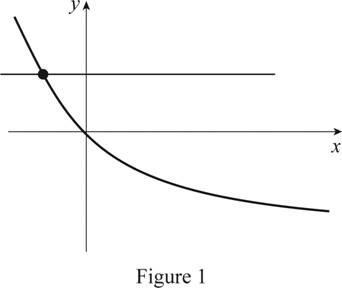# Whether the function given by a graph is one-to-one.### Single Variable Calculus: Concepts...

4th Edition
James Stewart
Publisher: Cengage Learning
ISBN: 9781337687805### Single Variable Calculus: Concepts...

4th Edition
James Stewart
Publisher: Cengage Learning
ISBN: 9781337687805

#### Solutions

Chapter 1.6, Problem 7E
To determine

## Whether the function given by a graph is one-to-one.

Expert Solution

The given function is one-to one.

### Explanation of Solution

Result used:

Horizontal line test states that the graph of the function is one-to-one function if and only if a horizontal line intersects the graph exactly once.

Calculation:

Perform the horizontal line test for the given graph.

Draw a horizontal line in such a way that it passes through the curve as shown in Figure 1.From Figure 1, it is observed that the horizontal line intersects the curve exactly at once, which means it passes the horizontal line test.

Therefore, the function given by the graph is a one-to one function.

### Have a homework question?

Subscribe to bartleby learn! Ask subject matter experts 30 homework questions each month. Plus, you’ll have access to millions of step-by-step textbook answers!